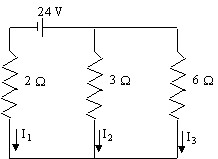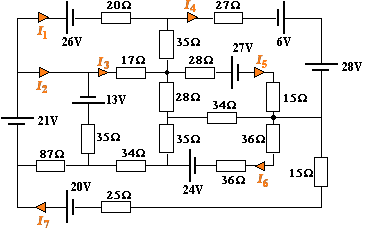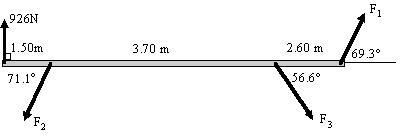Search IntMath
Close

# 6. Matrices and Linear Equations

by M. Bourne

We wish to solve the system of simultaneous linear equations using matrices:

a1x + b1y = c1
a2x + b2y = c2

If we let

A=((a_1,b_1),(a_2,b_2)), \ X=((x),(y))\  and \ C=((c_1),(c_2))

then AX=C. (We first saw this in Multiplication of Matrices).

If we now multiply each side of

AX = C

on the left by

A-1, we have:

A-1AX = A-1C.

However, we know that A-1A = I, the Identity matrix. So we obtain

IX = A-1C.

But IX = X, so the solution to the system of equations is given by:

X = A-1C

See the box at the top of Inverse of a Matrix for more explanation about why this works.

Note: We cannot reverse the order of multiplication and use CA-1 because matrix multiplication is not commutative.

### Example - solving a system using the Inverse Matrix

Solve the system using matrices.

x + 5y = 4

2x + 5y = −2

We have:

A=((-1,5),(2,5)),  \ X=((x),(y))\  and \ C=((4),(-2))

To solve the system, we need the inverse of A, which we write as A-1.

((5,5),(2,-1))

Change signs of the other 2 elements:

((5,-5),(-2,-1))

Now we find the determinant of A:

|A| = -5 - 10 = -15

So

A^-1 =-1/15((5,-5),(-2,-1))  = ((-1/3,1/3),(2/15,1/15))  = ((-0.333,0.333),(0.133,0.067))

So the solution to the system is given by:

X=A^-1C =((-0.333,0.333),(0.133,0.067))((4),(-2)) =((-2),(0.4))

This answer means that we have found the solution x = -2 and y = 0.4.

Is the solution correct?

We check it in the original set of equations:

{:(-x+5y,=4),(2x+5y,=-2):}

Substituting x = -2 and y = 0.4, we get:

−(−2) + 5×(0.4) = 2 + 2 = 4 [Checks OK]

2×(−2) + 5×(0.4)  = −4 + 2  = −2 [Checks OK]

So the solution to the original system of equations is

x = -2,\ \ y = 0.4.

## Solving 3×3 Systems of Equations

We can extend the above method to systems of any size. We cannot use the same method for finding inverses of matrices bigger than 2×2.

We will use a Computer Algebra System to find inverses larger than 2×2.

### Example - 3×3 System of Equations

Solve the system using matrix methods.

{: (x+2y-z=6),(3x+5y-z=2),(-2x-y-2z=4) :}

Did I mention? It's a good idea to always check your solutions.

A=((1,2,-1),(3,5,-1),(-2,-1,-2)), X=((x),(y),(z)), and C=((6),(2),(4))

Using Scientific Notebook, we find the inverse of A to be:

A^-1=((5.5,-2.5,-1.5),(-4,2,1),(-3.5,1.5,0.5))

(We could have used Gauss-Jordan Elimination if we need to show all steps.)

So the solution to the system of equations is:

X=A^-1C

=((5.5,-2.5,-1.5),(-4,2,1),(-3.5,1.5,0.5))((6),(2),(4))

=((22),(-16),(-16))

Check:

22 + 2(-16) - (-16) = 6 [Checks OK]

3(22) + 5(-16) - (-16) = 2 [Checks OK]

-2(22) - (16) - 2(-16) = 4 [Checks OK]

So the solution is x = 22, y = -16 and z = -16.

### Example - Electronics application of 3×3 System of Equations

Find the electric currents shown by solving the matrix equation (obtained using Kirchhoff's Law) arising from this circuit:((I_1+I_2+I_3),(-2I_1+3I_2),(-3I_2+6I_3))=((0),(24),(0))

(You can explore what the solution for this example really means in this 3D interactive systems of equations applet.)

We can write this as:

((1,1,1),(-2,3,0),(0,-3,6))((I_1),(I_2),(I_3))=((0),(24),(0))

So we have:

((I_1),(I_2),(I_3))=((1,1,1),(-2,3,0),(0,-3,6))^-1((0),(24),(0))

Using a computer algebra system to perform the inverse and multiply by the constant matrix, we get:

I_1= -6\ "A"

I_2= 4\ "A"

I_3= 2\ "A"

We observe that I1 is negative, as expected from the circuit diagram.

### Exercise 1

The following equations are found in a particular electrical circuit. Find the currents using matrix methods.

{: (I_A+I_B+I_C=0),(2I_A-5I_B=6),(5I_B-I_C=-3) :}

(This example is also included in the 3D interactive systems of equations applet.)

We need to form the matrices:

A=((1,1,1),(2,-5,0),(0,5,-1)), \ X=((I_A),(I_B),(I_C))\  and \ C=((0),(6),(-3))

Using Scientific Notebook (or any Computer Algebra System), we find:

A^-1  = ((0.294,0.353,0.294),(0.118,-0.059,0.118),(0.588,-0.294,-0.412))

and so (continuing to use Scientific Notebook, with rounding to 3 decimal places):

((I_A),(I_B),(I_C))=A^-1C

=((0.294,0.353,0.294),(0.118,-0.059,0.118),(0.588,-0.294,-0.412))((0),(6),(-3))

=((1.236),(-0.708),(-0.528))

Therefore

I_A= 1.236\ "A",

I_B= -0.708\ "A" and

I_C= -0.528\ "A"

### Exercise 2

Recall this problem from before? If we know the simultaneous equations involved, we will be able to solve the system using inverse matrices on a computer.The circuit equations, using Kirchhoff's Law:

−26 = 72I1 − 17I3 − 35I4

34 = 122I2 − 35I3 − 87I7

−4 = 233I7 − 87I2 − 34I3 − 72I6

−13 = 149I3 − 17I1 − 35I2 − 28I5 − 35I6 − 34I7

−27 = 105I5 − 28I3 − 43I4 − 34I6

24 = 141I6 − 35I3 − 34I5 − 72I7

5 = 105I4 − 35I1 − 43I5

What are the individual currents, I1 to I7?

### Phone usersNOTE: If you're on a phone, you can scroll any wide matrices on this page to the right or left to see the whole expression.

#### Solving currents in a Circuit (7 × 7 system)

We solve this using a computer as follows. We just write the coefficient matrix on the left, find the inverse (raise the matrix to the power -1) and multiply the result by the constant matrix.

You can use Matlab, Mathcad or similar math software to do this. Wolfram|Alpha is a free alternative.

X=[(72,0,-17,-35,0,0,0), (0,122,-35,0,0,0,-87), (0,-87,-34,0,0,-72,233), (-17,-35,149,0,-28,-35,-34), (0,0,-28,-43,105,-34,0), (0,0,-35,0,-34,141,-72), (-35,0,0,105,-43,0,0)]^-1 [(-26),(34),(-4),(-13),(-27),(24),(5)]

=[(-0.46801),(0.42932),(5.193xx10^-3),(-0.22243),(-0.27848),(0.21115),(0.20914)]

The answer means that the currents in this circuit are (to 4 decimal places):

I_1 = -0.4680\ "A"

I_2= 0.4293\ "A"

I_3= 0.0005\ "A"

I_4= -0.2224\ "A"

I_5= -0.2785\ "A"

I_6= 0.2112 \ "A"

I_7= 0.2091 \ "A"

### Exercise 3

We want 10 L of gasoline containing 2% additive. We have drums of the following:

We need to use 4 times as much pure gasoline as 5% additive gasoline. How much of each is needed?

Let

x = no. of litres of pure gasoline

y = no. of litres of 5% gasoline

z = no. of litres of 6% gasoline

From the first sentence, we have:

x + y + z = 10

The second sentence gives us:

We get NO additive from the pure gasoline.

We get (5% of y) L of additive from the second drum.

We get (6% of z) L of additive from the third drum.

We NEED 2% of 10 L of additive = 0.2 L = 200 mL.

So

0.05y + 0.06z = 0.2

Multiplying through by 100 gives us:

5y + 6z = 20

The second last sentence gives us:

x = 4y

We can write this as:

x - 4y = 0

This gives us the set of simultaneous equations:

x + y + z = 10

5y + 6z = 20

x − 4y = 0

So

A=((1,1,1),(0,5,6),(1,-4,0)), \ C=((10),(20),(0))

Using Scientific Notebook for the inverse:

((1,1,1),(0,5,6),(1,-4,0))^-1 =((0.96,-0.16,0.04),(0.24,-0.04,-0.24),(-0.2,0.2,0.2))

Multiplying the inverse by matrix C:

((0.96,-0.16,0.04),(0.24,-0.04,-0.24),(-0.2,0.2,0.2))((10),(20),(0)) =((6.4),(1.6),(2))

So we have 6.4 L of pure gasoline, 1.6 L of 5% additive and 2 L of 6% additive.

Is it correct?

6.4 + 1.6 + 2 = 10 L [Checks OK]

5% xx 1.6 + 6% xx 2 = 200 mL [Checks OK]

4 × 1.6 = 6.4 [Checks OK]

### Exercise 4

This statics problem was presented earlier in Section 3: Matrices.From the diagram, we obtain the following equations (these equations come from statics theory):

Vertical forces:

F1 sin 69.3° − F2 sin 71.1° − F3 sin 56.6° + 926 = 0

Horizontal forces:

F1 cos 69.3° − F2 cos 71.1° + F3 cos 56.6° = 0

Moments:

7.80 F1 sin 69.3° − 1.50 F2 sin 71.1° − 5.20 F3 sin 56.6° = 0

Using matrices, find the forces F1, F2 and F3.

We write the first equation so that the constant term is on the right hand side:

F1 sin 69.3° − F2 sin 71.1° − F3 sin 56.6° = −926

In matrix form, we write the equations as:

((sin 69.3°,-sin 71.1°,-sin 56.6°),(cos 69.3°,-cos 71.1°,cos 56.6°),(7.80 sin 69.3°,-1.50 sin 71.1°,-5.20 sin 56.6°))((F_1),(F_2),(F_3))

=((-926),(0),(0))

So the solution for the system is:

((F_1),(F_2),(F_3))=((sin 69.3°,-sin 71.1°,-sin 56.6°),(cos 69.3°,-cos 71.1°,cos 56.6°),(7.80 sin 69.3°,-1.50 sin 71.1°,-5.20 sin 56.6°))^-1((-926),(0),(0))

=((425.5),(1079.9),(362.2))

So

F_1= 425.5\ "N"

F_2= 1079.9\ "N"

F_3= 362.2\ "N"

This is very easy and quick in Scientific Notebook, Matlab or any other computer algebra system!

## Problem SolverThis tool combines the power of mathematical computation engine that excels at solving mathematical formulas with the power of GPT large language models to parse and generate natural language. This creates math problem solver thats more accurate than ChatGPT, more flexible than a calculator, and faster answers than a human tutor. Learn More.